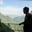Related Tags

r lists
r programming
data structure
communitycreator

# What are Lists in R?AKASH BAJWA

Lists are objects that contain elements of different types, such as:

• numbers
• strings
• vectors
• matrices
• dataframes
• functions
• nested listslists with other lists inside them

These can be created by using the list() function.

### Syntax

Note that these attributes attr1 and attr2 are the components of data structures such as numbers, strings, vectors, etc.

my_list <- list(attr1, attr2 ...)


### Creating a List

This is an example of how to create a list that contains elements like strings, numbers, vectors, and a logical value.

Illustration of below Example

### Example

The code below creates a list and prints each element of the list with the corresponding index.

#Create a list contain strings, numbers, vectors and a logical values
List_data <- list("Red", "Green", c(21,32, 11), TRUE, 51.23, 119.1)
print(List_data)


### 1. Modifying a component

We can modify a component in a list through reassignment by accessing a specific index and assigning this index with another new value.

//modifying the results
List_data[["Red"]] <- "Blue";


Adding a new component to the list is simple. Here is the basic syntax:

// adding new value
List_data[["White"]] <- FALSE


### 3. Deleting a component

Assigning FALSE to a specific index or value, it results in deletion.

//
List_data[["Red"]] <- NULL


RELATED TAGS

r lists
r programming
data structure
communitycreator

CONTRIBUTORAKASH BAJWA
RELATED COURSES

View all Courses

Keep Exploring

Learn in-demand tech skills in half the time